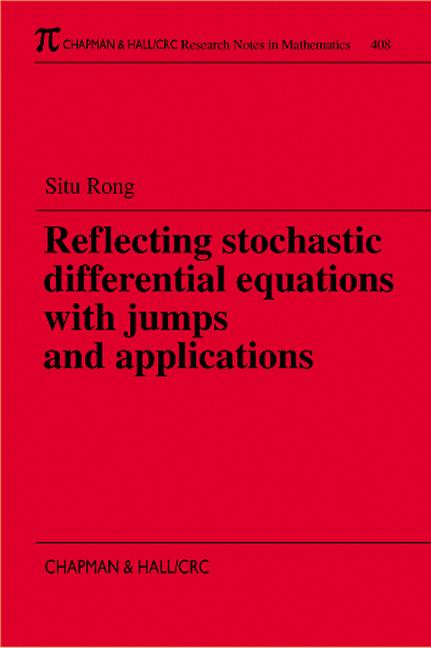# Reflecting Stochastic Differential Equations with Jumps and Applications

## 1st Edition

Chapman and Hall/CRC

224 pages

##### Purchasing Options:\$ = USD
Paperback: 9781584881254
pub: 1999-08-05
SAVE ~\$37.00
Currently out of stock
\$185.00
\$148.00
x

FREE Standard Shipping!

### Description

Many important physical variables satisfy certain dynamic evolution systems and can take only non-negative values. Therefore, one can study such variables by studying these dynamic systems. One can put some conditions on the coefficients to ensure non-negative values in deterministic cases. However, as a random process disturbs the system, the components of solutions to stochastic differential equations (SDE) can keep changing between arbitrary large positive and negative values-even in the simplest case. To overcome this difficulty, the author examines the reflecting stochastic differential equation (RSDE) with the coordinate planes as its boundary-or with a more general boundary.

Reflecting Stochastic Differential Equations with Jumps and Applications systematically studies the general theory and applications of these equations. In particular, the author examines the existence, uniqueness, comparison, convergence, and stability of strong solutions to cases where the RSDE has discontinuous coefficients-with greater than linear growth-that may include jump reflection. He derives the nonlinear filtering and Zakai equations, the Maximum Principle for stochastic optimal control, and the necessary and sufficient conditions for the existence of optimal control.

Most of the material presented in this book is new, including much new work by the author concerning SDEs both with and without reflection. Much of it appears here for the first time. With the application of RSDEs to various real-life problems, such as the stochastic population and neurophysiological control problems-both addressed in the text-scientists dealing with stochastic dynamic systems will find this an interesting and useful work.

### Reviews

"The author tries to obtain the weakest assumptions in his theorems, so this should be of interest to specialists who have to deal with systems with unusual conditions."

-J. Picard, Zentralblatt MATH Vol. 942

Promo Copy

SOME RECENT RESULTS ON SDE WITH JUMPS IN 1-DIMENSIONAL SPACE

Local Time and Occupation Density Formula

A Generalization of Ito's Formula

The Continuity of Local Time

Krylov Estimation

Tanaka Formula

Uniqueness of Solutions to Stochastic Differential Equations

Comparison for Solutions of Stochastic Differential Equations

Convergence of Solutions to Stochastic differential Equations

Existence of Solutions to Stochastic Differential Equations

Tanaka formula for SDE with Poisson Jumps in n-Dimensional Space

SKOROHOD PROBLEMS WITH GIVEN CADLAG FUNCTIONS

The Space D and Skorohod's Topology

Skorohod's Problem in a General Domain. Solution with Jumps

Skorohod Problem with Jump Reflection in a Half Space

REFLECTING STOCHASTIC DIFFERENTIAL EQUATIONS WITH JUMPS

Yamada-Watanabe Theorem, Tanaka Formula and Krylov Estimate

Moment Estimates and Existence of Solutions for Random Coefficients

Existence of Solutions for RSDE with Jumps

Existence of Solutions with Jump Reflection in a Half Space

PROPERTIES OF SOLUTIONS TO RSDE WITH JUMPS

Convergence Theorems for Solutions

Stability of Solutions

Comparison of Solutions

Applications of Comparison Theorem to 1-Dimensional RSDE

Uniqueness of Solutions

Convergence of Solutions in Half Space

NONLINEAR FILTERING OF RSDE

Representation of Martingales (Functional Coefficient Case)

Non-Linear Filtering Equation

Zakai Equation

STOCHASTIC CONTROL

Girsanov Theorem with Weak Conditions

Martingale Method, Necessary and Sufficient Conditions for Optimal Control

STOCHASTIC POPULATION CONTROL

Stochastic Population Control Model and Maximum Principle

Pathwise Stochastic Population Control and Stability of Population

Applications to Neurophysiological Control and Others

BIBLIOGRAPHY

### Subject Categories

##### BISAC Subject Codes/Headings:
MAT003000
MATHEMATICS / Applied
MAT007000
MATHEMATICS / Differential Equations